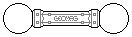Web textodigital.com
The giant cube
The giant cube can be built using either rhombitruncated cuboctahedra or rhombicosidodecahedra in place of the balls. In this case, we have chosen the reinforced version of the former (see a giant cube with rhombicosidodecahedra as vertices at the Atomium page). This model is built to scale 11.43, being 1 the normal, 12-rod, 8-ball cube.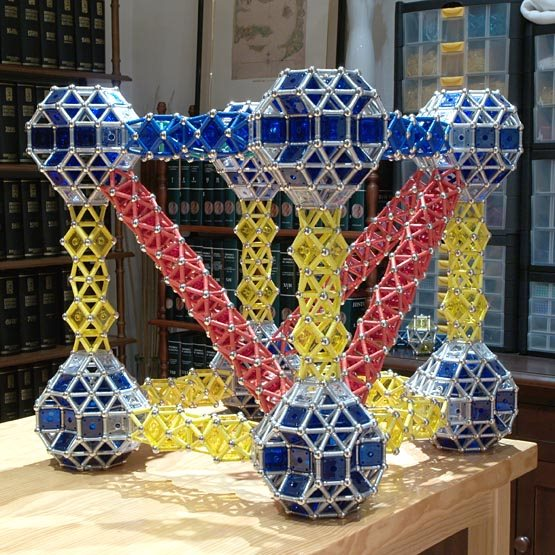Giant cube, view 1 6562 pieces: 1384 balls, 4200 rods, 978 squares (33.42 kg)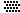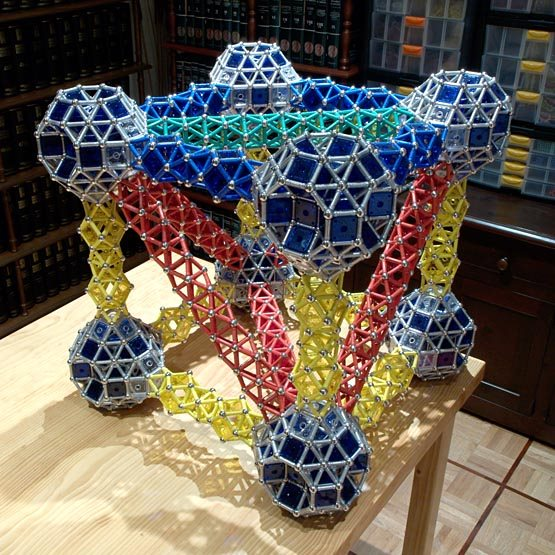Giant cube, view 2
The only challenge in building this cube was finding a design for the edges which allowed us to fit also face diagonals. So, the edges are built on the octagonal faces of the rhombitruncated cuboctahedron, and are made of two square cupolas, four square antiprisms, and two cuboctahedra. The face diagonals are built on square faces, and are made of 14 square antiprisms; this slightly exceeds (by less than 0.1%) the length that they should theoretically have.
The face diagonals included in this model form a regular tetrahedron standing on one of its edges, as shown in the following pictures. The scale of this tetrahedron is 16.19: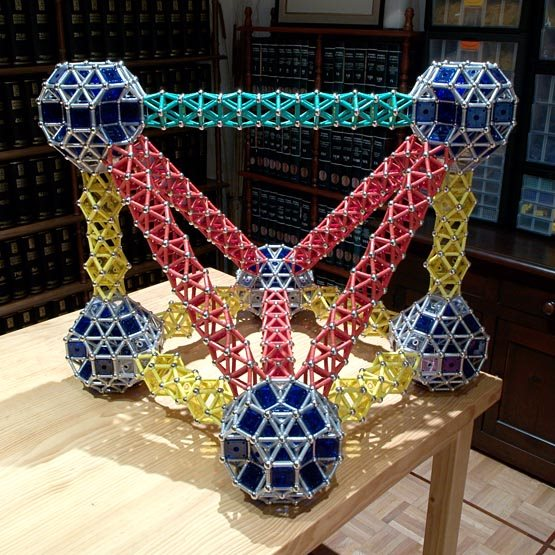Giant tetrahedron in the giant cube, view 1 4764 pieces: 1008 balls, 3072 rods, 684 squares (24.35 kg)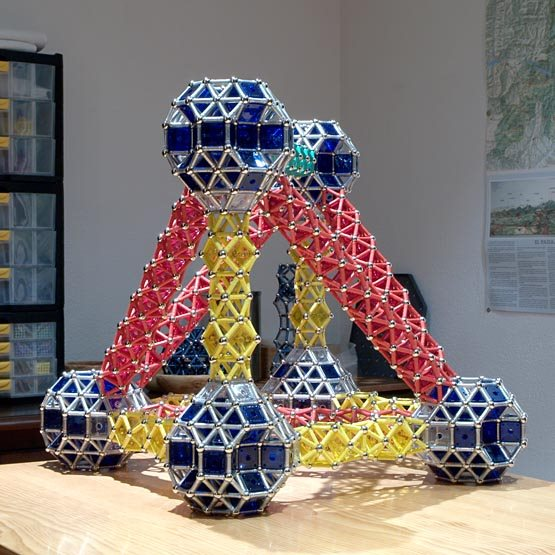Giant tetrahedron in the giant cube, view 2